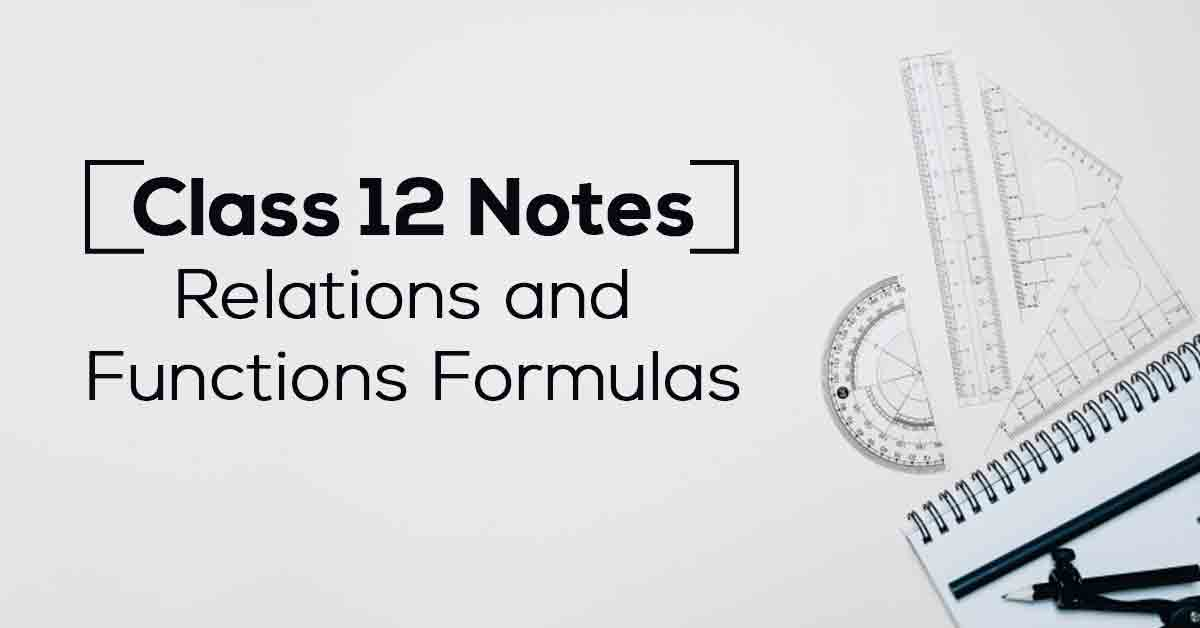Class 12th Math Relations And Functions Formulas CBSE 2023

# Relations and Functions Class 12 Notes# Chapter 1 Relations and Functions Formulas

NCERT Year 12 Math notes provide a solid conceptual foundation for all topics covered in the CBSE Year 12 Math Program, detailing important theorems and formulas to help students better understand concepts. Vidyakul's NCERT solution can help you prepare for your Year 12 exam.

Vidyakul’s Year 12 Math experts have compiled all NCERT Year 12 math notes in this article. It has over 32 topics in each math textbook, illustrated by top academic experts from around the world. Students who want to do well in their upcoming exams should turn to the Vidyakul learning platform for excellent results. Read on to learn more and access all the NCERT Class 12 notes you need.

### Point to Remember

Below we have provided some of the important points to remember for NCERT Class 12 Maths notes to ace the exams:

• Relations and Functions are an essential part of Mathematics because they help define various concepts and different types of specific valued functions and their graphs.

• The term ‘relation’ in Mathematics has been drawn from the meaning of relationships in the English language, according to which two objects or quantities are related if there is a recognizable connection or link between the two objects or quantities.

• Inverses of trigonometric functions exist solely because of the constraints on the domains and their respective ranges.

• The inverse trigonometric functions play an essential role in calculus, for they serve to define many integrals. The concepts of inverse trigonometric functions are used in science and engineering.

• A matrix is an array of numbers or functions arranged in a rectangular pattern.

• The determinant is a scalar value that can be measured from the elements of a square matrix and encodes certain properties of the linear transformation described by the matrix. The determinant of a matrix A is denoted det(A), det A, or |A|.

• The real value at a point in a function is said to be continuous when the function of that point is equal to the limit of the function at that point. When the entire domain is continuous, there is continuity.

• Differential Calculus is centered on the concept of the derivative. Integral Calculus is motivated by the problem of defining and calculating the region’s area bounded by the graph of the functions.

• Integration refers to the process that is the inverse of differentiation. We will be responsible for finding the function whose differential is provided to us during the integration.

• Differential equations involve the derivative of the dependent variable concerning the independent variable.

• Every identity function runs indefinitely. Every continuous function is a constant function. Every polynomial function is a continuous function. Every rational function runs indefinitely. In their domain, all trigonometric functions are continuous.

• Linear programming is the process of optimizing problems that are constrained in some way.

• The likelihood of an event or outcome occurring based on a previous event or outcome is conditional probability.

• An event or a case is a subset of the sample space associated with a random experiment. For example, flipping a coin and getting either head or tail is an event.

### Topics and Sub-topicsSr NoTopic Name1.1Types of Relations1.2Types of Functions1.3Composition of Functions1.4Invertible Functions1.5Binary Operations

Download the FREE PDF of Relations and Functions Class 12 Notes and start your preparation with Vidyakul!​